# Electronics and Communication Engineering - Power Electronics

Exercise : Power Electronics - Section 1
41.
The number of leads in an SCR are :
2
3
4
5
Explanation:

Anode, cathode and gate.

42.
In a single phase full wave converter (M-2 connection) feeding on R - L load, the input voltage is v = Vm sin ωt. The expression for dc output voltage is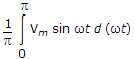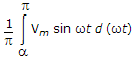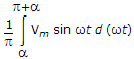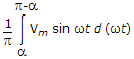Explanation:

Due to inductive load the period of conduction is a to p + a in each half cycle.

43.
A thyristor has a turn on time of 6 μs. If the anode circuit is inductive, the turn on time will be
6 μs
less than 6μs
more than 6 μs
either 6 μs or less
Explanation:

Inductive load increases the turn on time.

44.
The total number of leads in reverse conducting thyristor is
2
3
1
4
Explanation: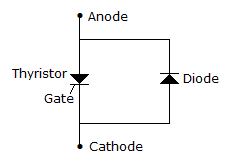45.

Assertion (A): A TRIAC is a bidirectional SCR.

Reason (R): A TRIAC is a four layers 3 terminal device and can conduct in both directions.

Both A and R are correct and R is correct explanation of A
Both A and R correct but R is not correct explanation of A
A is correct but R is wrong
A is wrong but R is correct